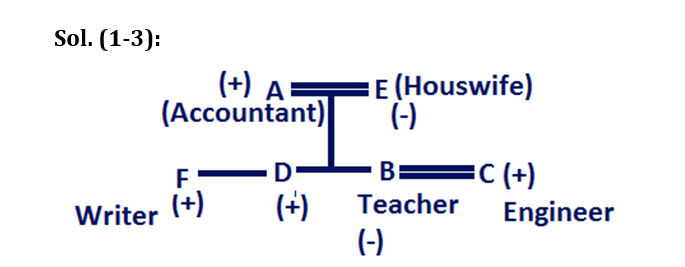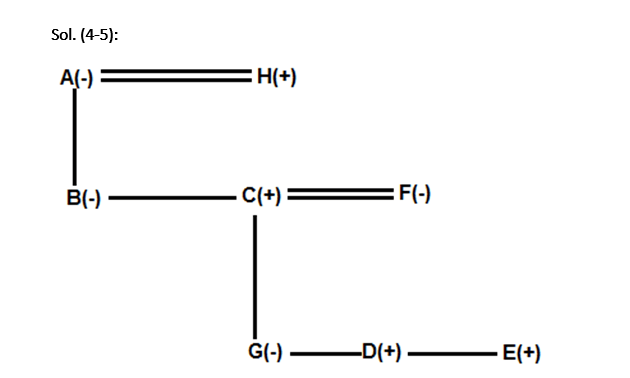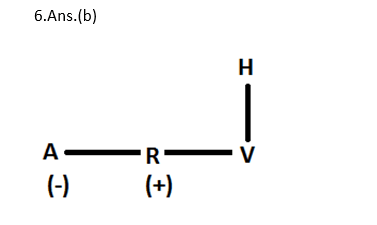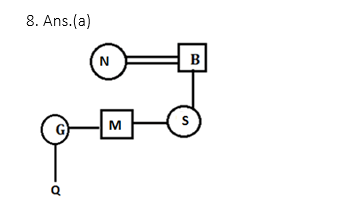# Blood relation for IBPS, SBI, RBI, RRBs: Quiz 1

## Blood relation for IBPS, SBI, RBI, RRBs: Quiz 1

Blood relation plays a significant role in Reasoning Ability Section of banking exams such as IBPS, SBI and RBI PO and Clerk. You will get at least 5 questions from Blood relation in one of IBPS, SBI and RBI PO & clerk prelims and Mains exam. So, aspirants should focus on Blood relation questions in detail. Here, we are providing you with the Blood relation questions quiz with the detailed solution so that you can easily prepare for Blood relation questions. We are providing here all-important latest pattern-based questions and Previous Year Questions of Blood relation of various Government Exam like IBPS, SBI, and RBI PO and Clerk exam. This Blood relation quiz we are providing is free. Attempt this Blood relation quiz to practice important questions with answers and solutions. And score better in IBPS, SBI and RBI PO and Clerk exam.

Blood relation Quiz to improve your Reasoning Ability for SBI Po & SBI clerk exam IBPS PO Reasoning, IBPS Clerk Reasoning, IBPS RRB Reasoning, LIC AAO, LIC Assistant and another competitive exam.

Questions (1-3): Study the following information and answer the questions that follow:

There are six members i.e. A, B, C, D, E and F in a family having two generation. There are two married couple in the family. They all have different profession, only D has no profession. The different professions are teacher, Accountant, Engineer, Housewife and Writer but not necessarily in the same order. F is the son of E, who is a housewife. B is teacher and has only two brothers. C is an Engineer and is son in law of A, who is an Accountant. D is not the female member of the family nor a Writer.

Q1. Who are the two female members in the family?

(a) A and E

(b) B and D

(c) E and F

(d) E and B

(e) None of these1.Ans.(d)

Q2. What is the profession of the wife of C?

(a) Writer

(b) Teacher

(c) Accountant

(d) Engineer

(e) She is a housewife2.Ans.(b)

Q3. Who is the Father B?

(a) C

(b) D

(c) The one who is Writer

(d) None of these

(e) The one who is Accountant3.Ans.(e)

Directions (4-5): Study the given information carefully and answer the given questions.

H has only two children-B and C. B is the sister of C. D is the son of C. E is the brother of D. F is the mother of E. G, who is sister of D, is the granddaughter of A who is the mother of B.

Q4. How is A related to E?

(a)  Grandmother

(b)  Son

(c)   Mother

(d)  Cousin Brother

(e)   None of these1. Ans.(a)

Q5. How is G related to A’s daughter in law?

(a)  Sister

(b)  Mother

(c)  Son

(d)  Daughter

(e)  None of these1. Ans.(d)

Directions (6-7): Study the following information carefully and answer the questions that follow:

A ÷ B means A is son of B

A × B means A is sister of B

A + B means A is brother of B

A – B means A is mother of B

Q6. How is A related to H in the expression ‘A × R + V ÷ H’?

(a) Sister

(b) Daughter

(c) Son

(d) Mother

(e) None of theseQ7. Which of the following expressions represents ‘D is the husband of M’?

(a) M × R – S + D

(b) M – R + S ÷ D

(c) M + R ÷ S × D

(d) M ÷ R × S + D

(e) None of theseDirections (8-10): Each of these questions is based on the following information:

(i) Y % Z means Y is the daughter of Z.

(ii) Y @ Z means Y is the mother of Z.

(iii) Y \$ Z means Y is the father of Z.

(iv) Y * Z means Y is the son of Z.

Q8. Which of the following expression shows the relation that B is father of G?

(a) S % B \$ M * N@ G @ Q

(b) B * F \$ G @ N@ M \$ D

(c) M @B * S * F @ G % D

(d) M @ B @ N @ S*G \$ D

(e) None of theseQ9. If the expression P%Y@G*K*F is definitely true, then which of the following is not true?

(a) G is son of F’s son

(b) K is husband of P’s mother

(c) P is daughter of K

(d) None of these

(e) F is father of YQ10. If the expression A % R @ C % B *E is definitely true, then which of the following is true?

(a) C is son of E

(b) E is father of B

(c) R is wife of B

(d) None of these

(e) A is brother of CBlood Relation Quiz-1 PDF

#### Aatma Nirbhar Reasoning Topic Wise Online Test Series

Recommended PDF’s for 2021:

### 2021 Preparation Kit PDF

#### Most important PDF’s for Bank, SSC, Railway and Other Government Exam : Download PDF Now

AATMA-NIRBHAR Series- Static GK/Awareness Practice Ebook PDF Get PDF here
The Banking Awareness 500 MCQs E-book| Bilingual (Hindi + English) Get PDF here
AATMA-NIRBHAR Series- Banking Awareness Practice Ebook PDF Get PDF here
Computer Awareness Capsule 2.O Get PDF here
AATMA-NIRBHAR Series Quantitative Aptitude Topic-Wise PDF 2020 Get PDF here
Memory Based Puzzle E-book | 2016-19 Exams Covered Get PDF here
Caselet Data Interpretation 200 Questions Get PDF here
Puzzle & Seating Arrangement E-Book for BANK PO MAINS (Vol-1) Get PDF here
ARITHMETIC DATA INTERPRETATION 2.O E-book Get PDF here

3